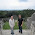## Arduino Controlled V-Amp Pro - Part 5

Extending the functionality of my hack job is necessary. I mean, when was the last time you saw someone spend hours building something and just wanted to hit them when they stopped at just good enough? I have some parts on order from the good folks at Sparkfun Electronics for phantom power and panel mount USB hookup (w00t!) and also a little surprise that I will be adding in as soon as I figure out how to mount it.

I had a thought the other day. If I can control volume and wah with the expression pedals, what else can I control? According to the manual (page 17) I can control quite a few things with them (not all are useful though). Then it was just a matter for code and how to shift between modes of operation.

Here is my logic: if you step on two of the effects pedals at once, the program sends the expression pedals into a different mode depending on which two were pressed.

First off, there has to be a global array variable that can be changed depending on what mode is desired. I put “int expression_choice[] = {1,2};” at the top of the code. I arbitrarily assigned wah the value of one and volume the value of two. Array index 0 is on the left and index 1 is on the right for left and right pedals respectively.

Secondly, there has to be something in the loop() function to catch when the two buttons are pressed simultaneously. For this it is necessary to put it BEFORE the effects change buttons in the if statement within the loop(). Here's what I did in order to get Volume/Wah, Double Wah, Reverb/Volume and Delay/Volume.
``` void loop() {   //////////////////////   //Expression Options//   //////////////////////   if (digitalRead(23) == LOW && digitalRead(25) == LOW)//1 AND 2   {     expression_choice = 1;  //Wah     expression_choice = 2;  //Volume   }   else if (digitalRead(25) == LOW && digitalRead(29) == LOW)//2 AND 3   {     expression_choice = 1;  //Wah     expression_choice = 1;  //Double Wah!   }   else if (digitalRead(29) == LOW && digitalRead(27) == LOW)//3 AND 4   {     expression_choice = 3;  //Reverb     expression_choice = 2;  //Volume   }   else if (digitalRead(27) == LOW && digitalRead(33) == LOW)//4 AND 5   {     expression_choice = 4;  //Delay     expression_choice = 2;  //Volume   }   ///////////   //EFFECTS//   ///////////   //   //Lower Button Bank   //   //Button 1   else if(digitalRead(23) == LOW).... ```

Beyond that, I did a bit of a shakeup at the bottom of the code. Since the left and right pedals are no longer strictly wah and volume, I renamed the changeVolume and wahCharacter functions to reflect that. Also in those functions, I added some extra if logic so that based on what value expression_choice is it will reflect that by sending the proper Control Change codes for whatever mode I want. Here's the bottom of the code.
``` void rightPedal(byte channel, byte volume) {   Serial.print(0xB0 | (channel & 0x0F), BYTE); //Control Change   if(expression_choice == 2){     Serial.print(0x07, BYTE);//Volume CC number   }   else if(expression_choice == 1)   {     Serial.print(0x1B, BYTE);//Wah character CC number   }   Serial.print(volume & 0x7F, BYTE);//Value (0-127) } void leftPedal(byte channel, byte wah) {   Serial.print(0xB0 | (channel & 0x0F), BYTE);//Control Change   if(expression_choice == 1)   {     Serial.print(0x1B, BYTE);//Wah character CC number   }   else if(expression_choice == 3)   {     Serial.print(0x12, BYTE);//Reverb character CC number   }   else if(expression_choice == 4)   {     Serial.print(0x32, BYTE);//Delay timing CC number   }   Serial.print(wah & 0x7F, BYTE);//Value (0-127) } void expression(int pin) {   static int s_nLastPotValue = 0;   static int s_nLastMappedValue = 0;   int nCurrentPotValue = analogRead(pin);   if(abs(nCurrentPotValue - s_nLastPotValue) < pot_threshold)     return;   s_nLastPotValue = nCurrentPotValue;   int nMappedValue = map(nCurrentPotValue, 0, 1023, 0, 127); // Map the value to 0-127   if(nMappedValue == s_nLastMappedValue)     return;   s_nLastMappedValue = nMappedValue;   if(nMappedValue >= 10)   {     if (pin == volume_pin)     {      rightPedal(midi_channel, nMappedValue);     }     else     {       leftPedal(midi_channel, nMappedValue);     }   }   else//Turn it off   {     if (pin == volume_pin)     {      rightPedal(midi_channel, 0);     }     else     {       leftPedal(midi_channel, 0);     }   } } ```

So if all goes according to plan, you should have something that works like this:
Categories: , , , ,

1.This the direction I'm going too. I'm documenting my project at www.practicalusage.com
I will be using my FCB1010 and another pedal board to control a multi effect pedal board (Digitech GNX3000) and a Mac running Apple Logic/Mainstage, plus control physical interaction with various hardware.

2.It's just a matter of knowing what MIDI command to throw at what. This has been tested working with just about anything that has MIDI IN.

3.Hey - this is the sort of thing I want to do - my fcb1010 has just given up too :-(

I was lookiing at making a cusatom audio electronics version - making my own enclosure so i can have presets like on the fcb1010 but then a bank of stomp boxes at the top about 10 to control my axe fx sending cc messages. would i need 2 units and could they be connected together?

4.Are you talking about using it like an odd Channel Selector?

5.sort of -

the idea would be to have maybe 5 presets wich would pre select the patch (clean or dirty) as well as the effects within my saved patch.(example - if i had a clean patch my chorus would be automatically selected - there would also be a switch that allowed me to turn the chorus effect only off if i wanted to) now this patch might also contain several other effects (9 others) so i would have 15 buttons on my pedalboard to control preset changes and to toggle individual effects on and off. if that makes sense?

6.I've been having a play with this, have it all wired up but the midi is not sending, using midi ox to monitor it.

do you know the code for sending a cc message over to toggle a effect on n off?

Baz